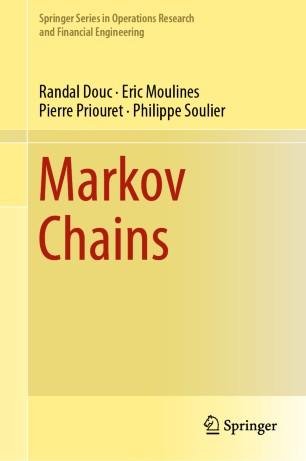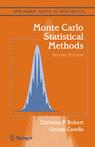## Markov Chains [not a book review]

Posted in Books, pictures, Statistics, University life with tags , , , , , , , , , , , , , on January 14, 2019 by xi'anAs Randal Douc and Éric Moulines are both very close friends and two authors of this book on Markov chains,  I cannot engage into a regular book review! Judging from the table of contents, the coverage is not too dissimilar to the now classic Markov chain Stochastic Stability book by Sean Meyn and the late Richard Tweedie (1994), called the Bible of Markov chains by Peter Glynn, with more emphasis on convergence matters and a more mathematical perspective. The 757 pages book also includes a massive appendix on maths and probability background. As indicated in the preface, “the reason [the authors] thought it would be useful to write a new book is to survey some of the developments made during the 25 years that have elapsed since the publication of Meyn and Tweedie (1993b).” Connecting with the theoretical developments brought by MCMC methods. Like subgeometric rates of convergence to stationarity, sample paths, limit theorems, and concentration inequalities. The book also reflects on the numerous contributions of the authors to the field. Hence a perfect candidate for teaching Markov chains to mathematically well-prepared. graduate audiences. Congrats to the authors!

## Gibbs for incompatible kids

Posted in Books, Statistics, University life with tags , , , , , , , , , , on September 27, 2018 by xi'anIn continuation of my earlier post on Bayesian GANs, which resort to strongly incompatible conditionals, I read a 2015 paper of Chen and Ip that I had missed. (Published in the Journal of Statistical Computation and Simulation which I first confused with JCGS and which I do not know at all. Actually, when looking at its editorial board,  I recognised only one name.) But the study therein is quite disappointing and not helping as it considers Markov chains on finite state spaces, meaning that the transition distributions are matrices, meaning also that convergence is ensured if these matrices have no null probability term. And while the paper is motivated by realistic situations where incompatible conditionals can reasonably appear, the paper only produces illustrations on two and three states Markov chains. Not that helpful, in the end… The game is still afoot!

## Gibbs for kidds

Posted in Books, Kids, Statistics, University life with tags , , , , , , , , , , , , , , , on February 12, 2018 by xi'anA chance (?) question on X validated brought me to re-read Gibbs for Kids, 25 years after it was written (by my close friends George and Ed). The originator of the question had difficulties with the implementation, apparently missing the cyclic pattern of the sampler, as in equations (2.3) and (2.4), and with the convergence, which is only processed for a finite support in the American Statistician paper. The paper [which did not appear in American Statistician under this title!, but inspired an animal bredeer, Dan Gianola, to write a “Gibbs for pigs” presentation in 1993 at the 44th Annual Meeting of the European Association for Animal Production, Aarhus, Denmark!!!] most appropriately only contains toy examples since those can be processed and compared to know stationary measures. This is for instance the case for the auto-exponential model$f(x,y) \propto exp(-xy)$

which is only defined as a probability density for a compact support. (The paper does not identify the model as a special case of auto-exponential model, which apparently made the originator of the model, Julian Besag in 1974, unhappy, as George and I found out when visiting Bath, where Julian was spending the final year of his life, many years later.) I use the limiting case all the time in class to point out that a Gibbs sampler can be devised and operate without a stationary probability distribution. However, being picky!, I would like to point out that, contrary, to a comment made in the paper, the Gibbs sampler does not “fail” but on the contrary still “converges” in this case, in the sense that a conditional ergodic theorem applies, i.e., the ratio of the frequencies of visits to two sets A and B with finite measure do converge to the ratio of these measures. For instance, running the Gibbs sampler 10⁶ steps and ckecking for the relative frequencies of x’s in (1,2) and (1,3) gives 0.685, versus log(2)/log(3)=0.63, since 1/x is the stationary measure. One important and influential feature of the paper is to stress that proper conditionals do not imply proper joints. George would work much further on that topic, in particular with his PhD student at the time, my friend Jim Hobert.

With regard to the convergence issue, Gibbs for Kids points out to Schervish and Carlin (1990), which came quite early when considering Gelfand and Smith published their initial paper the very same year, but which also adopts a functional approach to convergence, along the paper’s fixed point perspective, somehow complicating the matter. Later papers by Tierney (1994), Besag (1995), and Mengersen and Tweedie (1996) considerably simplified the answer, which is that irreducibility is a necessary and sufficient condition for convergence. (Incidentally, the reference list includes a technical report of mine’s on latent variable model MCMC implementation that never got published.)

## lemma 7.3

Posted in Statistics with tags , , , , , , , , , , , on November 14, 2012 by xi'anAs Xiao-Li Meng accepted to review—and I am quite grateful he managed to fit this review in an already overflowing deanesque schedule!— our 2004 book  Monte Carlo Statistical Methods as part of a special book review issue of CHANCE honouring the memory of George thru his books—thanks to Sam Behseta for suggesting this!—, he sent me the following email about one of our proofs—demonstrating how much efforts he had put into this review!—:

```I however have a question about the proof of Lemma 7.3
on page 273. After the expression of
E[h(x^(1)|x_0], the proof stated "and substitute
Eh(x) for h(x_1)".  I cannot think of any
justification for this substitution, given the whole
purpose is to show h(x) is a constant.```

I put it on hold for a while and only looked at it in the (long) flight to Chicago. Lemma 7.3 in Monte Carlo Statistical Methods is the result that the Metropolis-Hastings algorithm is Harris recurrent (and not only recurrent). The proof is based on the characterisation of Harris recurrence as having only constants for harmonic functions, i.e. those satisfying the identity$h(x) = \mathbb{E}[h(X_t)|X_{t-1}=x]$

The chain being recurrent, the above implies that harmonic functions are almost everywhere constant and the proof steps from almost everywhere to everywhere. The fact that the substitution above—and I also stumbled upon that very subtlety when re-reading the proof in my plane seat!—is valid is due to the fact that it occurs within an integral: despite sounding like using the result to prove the result, the argument is thus valid! Needless to say, we did not invent this (elegant) proof but took it from one of the early works on the theory of Metropolis-Hastings algorithms, presumably Luke Tierney’s foundational Annals paper work that we should have quoted…

As pointed out by Xiao-Li, the proof is also confusing for the use of two notations for the expectation (one of which is indexed by f and the other corresponding to the Markov transition) and for the change in the meaning of f, now the stationary density, when compared with Theorem 6.80.

## A slice of infinity

Posted in R, Statistics, University life with tags , , , , , , , on July 28, 2011 by xi'an

Peng Yu sent me an email about the conditions for convergence of a Gibbs sampler:

The following statement mentions convergence. But I’m not familiar what the regularity condition is.

“But it is necessary to have a finite probability of moving away from the current state at all times in order to satisfy the regularity conditions on which the whole MCMC theory depends.”

Slice sampler is discussed in your book Monte Carlo Statistical Methods. I think that the “regularity condition” may have been discussed in your book. If so, would you please let me know where it is? Thanks and look forward to hearing from you!

The quote is from Martyn Plummer and deals with a stopping rule in JAGS implementation of the slice sampler. (The correct wording should be “strictly positive probability” rather than “finite probability”, I think.) However, this has nothing to do with a “regularity condition” on the irreducibility of a Markov chain: if a slice sampler is implemented for an unbounded density target, say a Beta(1/2,1/2), there is no irreducibility condition connected with the infiniteness of the density. In theory, (a) the chain never visits the “state” where the density is infinite (if only because we are dealing with a continuous state space) and (b) after visiting a value x with a large density f(x), the slice sampler allows for a move away from it since the slice involves a uniform simulation over (0,f(x)). Deeper properties of the slice sampler (like geometric ergodicity) are explored in, e.g., this JRSS B paper by Gareth Roberts and Jeff Rosenthal and this one in the Annals of Statistics by Radford Neal. In practice, the problem is caused by values of f(x) that cannot be computed and hence produce an error message like

`Singularity in likelihood found by Slicer.`

If those singularities can be localised, a neighbourhood excluding them should be introduced. (More easily said than done, obviously!)

Here is an example of a slice sampler with the Beta(1/2,1/2) distribution:

```#graphics
dote=function(x,y) points(x,y,col="gold",pch=19,cex=.4)
mote=function(x,y,z,w) lines(c(x,z),c(y,w),col="gold",lwd=.5)
cst=dbeta(.5,.5,.5)*.5 #normalising constant
#inverting f(x)=d, 2nd degree equation
hitden=function(d) .5+.5*sqrt(1-4*( cst/ max(d,dbeta(.5,.5,.5)))^2)*c(-1,1)
#output
curve(dbeta(x,.5,.5),0,1,ylab="density",lwd=2,col="steelblue",n=1001)
x=runif(1);u=runif(1)*dbeta(x,.5,.5);dote(x,u)
for (t in 1:100){ #100 slice steps
bo=hitden(u)
nx=sample(c(runif(1,0,bo),runif(1,bo,1)),1)
nu=runif(1)*dbeta(nx,.5,.5)
mote(x,u,nx,nu)
x=nx;u=nu;dote(x,u)
}
```

which clearly explores the whole area under the Beta(1/2,1/2) density. Even when started at a large density value like f(.999999), it eventually leaves the vicinity of this highly improbable value.